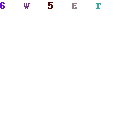Sharpening an image is merely the inverse of what we did to blur the image. It also uses the convolution function, which you can read in my post about Smoothing using convolution.

Here we supply a convolution matrix set up as follows:

 0 -2 0 -2 11 -2 0 -2 0

Here our weighting factor would total 3, since the negative numbers get subtracted from the weighting total.Shapening our image

You can download the full code for the sample application which contains code for all the image effects covered in the series here.

```public void ApplySharpen(double weight)
{
ConvolutionMatrix matrix = new ConvolutionMatrix(3);
matrix.SetAll(1);
matrix.Matrix[0, 0] = 0;
matrix.Matrix[1, 0] = -2;
matrix.Matrix[2, 0] = 0;
matrix.Matrix[0, 1] = -2;
matrix.Matrix[1, 1] = weight;
matrix.Matrix[2, 1] = -2;
matrix.Matrix[0, 2] = 0;
matrix.Matrix[1, 2] = -2;
matrix.Matrix[2, 2] = 0;
matrix.Factor = weight - 8;
bitmapImage = Convolution3x3(bitmapImage, matrix);

}
```